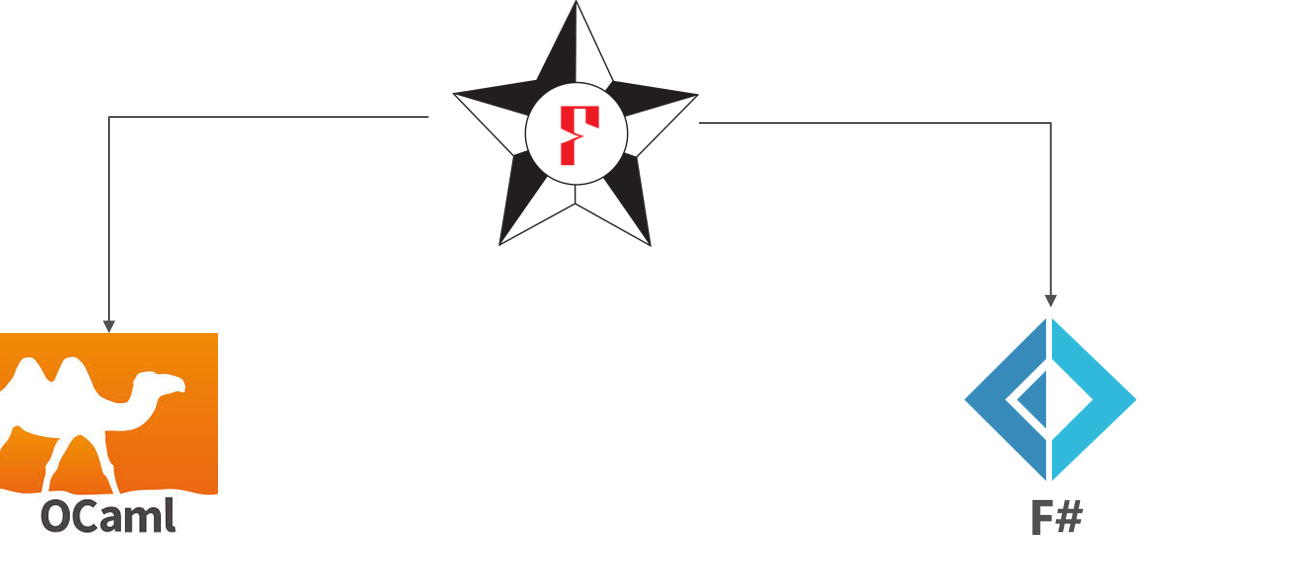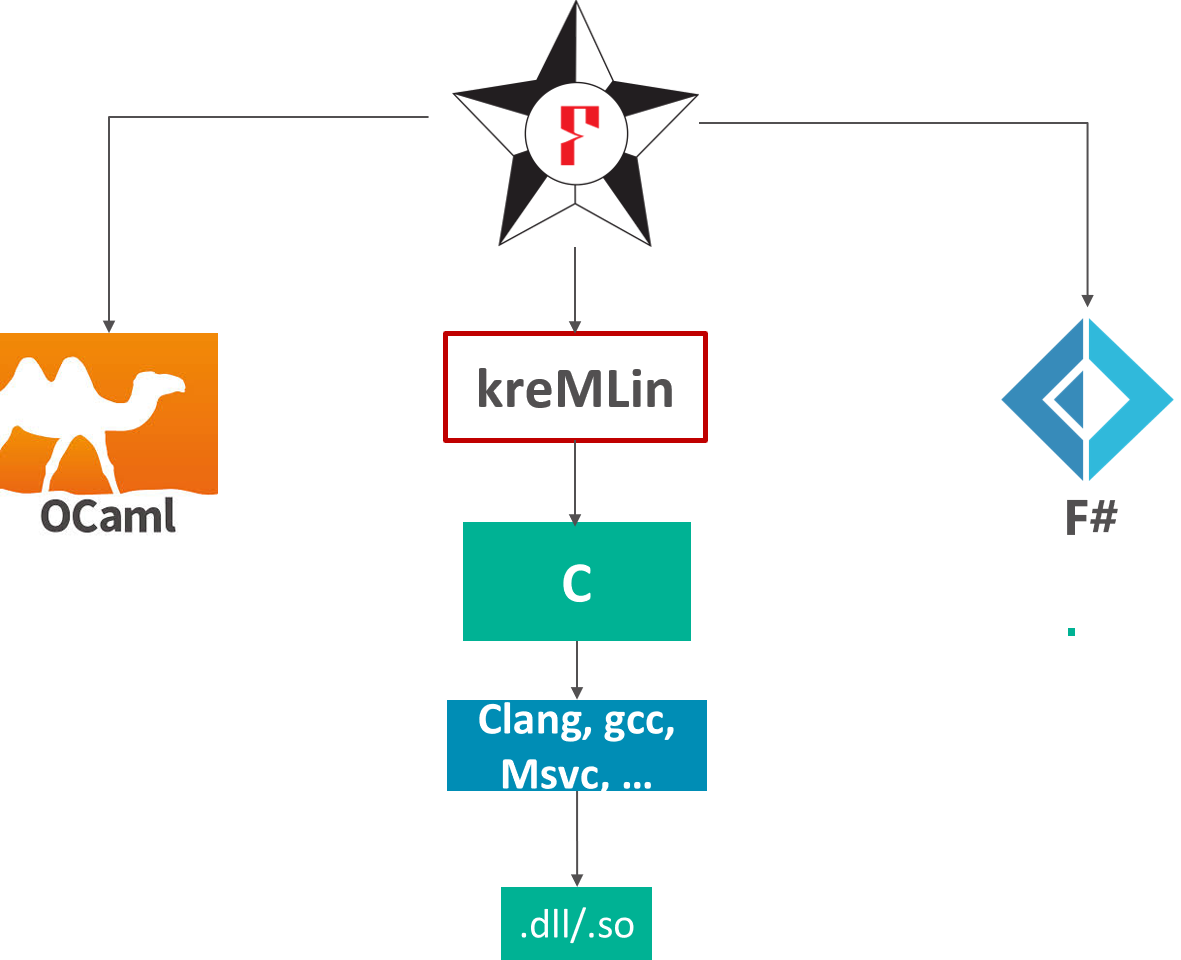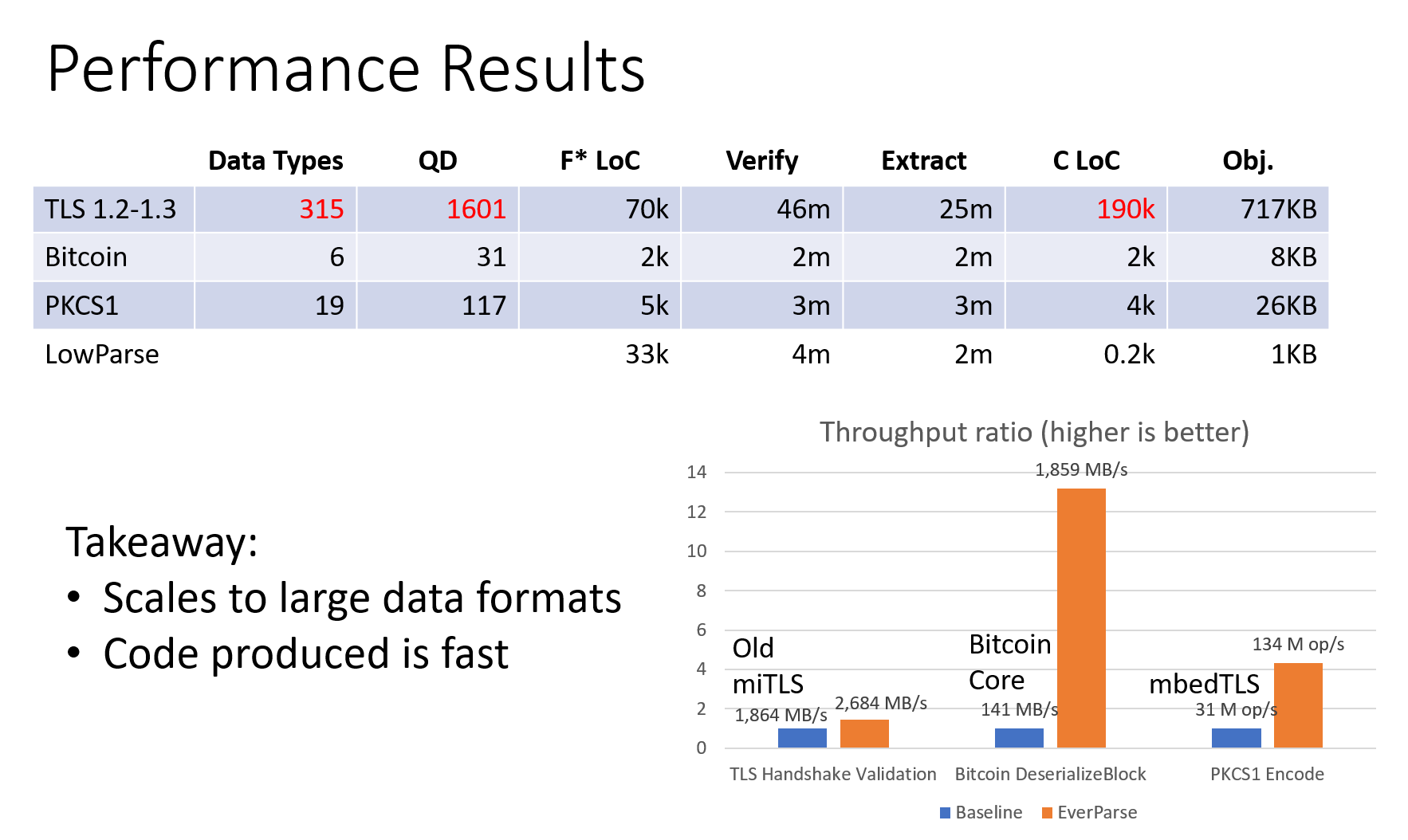## Verifying Imperative Programs with Functional Programming

Vancouver, BC

June 12, 2019

### Program verification: Shall the twain ever meet?

Interactive proof assistants Semi-automated verifiers of imperative programs
Coq, CompCert,   air Dafny, Verve,
Agda, Bedrock, Why3 miTLS
Lean, PVS, 4 colors   gap Vale
• In the left corner: Very expressive dependently-typed logics,
but only purely functional programming

• In the right: effectful programming, SMT-based automation,
but only first-order logic

### Bridging the gap: F*

• Functional programming language with effects

• F* extracted to OCaml or F# by default
• Subset of F* compiled to efficient C code
• Semi-automated verification system using SMT

• like Dafny, FramaC, Why3,
• With an expressive core language based on dependent type theory

• like Coq, Lean, Agda, PVS,
• A metaprogramming and tactic framework for interactive proof and user-defined automation

• like Coq, Isabelle, Lean, PVS, etc.

### A first taste

• Write ML-like code

let rec factorial n =
if n = 0 then 1
else n * factorial (n - 1)
• Give it a specification, claiming that factorial is a total function from non-negative to positive integers.

val factorial: n:int{n >= 0} -> Tot (i:int{i >= 1}) 
• Ask F* to check it

fstar factorial.fst
Verified module: Factorial
All verification conditions discharged successfully
fstar factorial.fst
Verified module: Factorial
All verification conditions discharged successfully
##### F* builds a typing derivation of the form:

• In a context including definitions of F* primitives

• The program has type , given the validity of a logical formula

• is passed to Z3 (an automated theorem prover/SMT solver) to check for validity

• If the check succeds, then, from the metatheory of F*, the program is safe at type

## Running F* Programs

#### Extraction to OCaml and FSharp## Running F* Programs

#### Extraction of a subset of F* to C### Some uses of F*

• Project Everest: verify and deploy new, efficient HTTPS stack
• miTLS: Verified reference implementation of TLS (1.2 and 1.3)
• EverParse: Verified parsers and formatter generators
• EverCrypt: Agile Cryptographic Provider
• HACL*: High-Assurance Cryptographic Library
• Vale: Verified Assembly Language for Everest
• Verified Everest code deployed in
• Firefox (Mozilla NSS crypto)
• Windows (WinQUIC)
• Azure Confidential Consortium (Verified Merkle tree for the blockchain)
• WireGuard VPN
• Zinc crypto library for Linux
• Tezos and Concordium blockchains

## The Current Everest and F* team

Microsoft Research, Inria Paris, CMU, MIT, Rosario, …

• many former members, interns, external contributors, you?

### This talk

• Need to write low-level code for performance

• Cryptographic routines are heavily optimized in C and assembly
• Networking protocols are inherently effectful
• Need to write low-level code for security

• Hard to control side-channels with a GC, JIT etc.
• Specifying and verifying effectful programs is hard

• Much effort involved in designing and structuring specifications, perhaps more effort than programming effectful code itself

• Typical code to proof ratio for functional correctness and security proofs is ~ 1 : 5 (more like 1 : 20 without SMT)

• Pays to structure specifications well functional programming can help

• Functional programming ~ Freedom of abstraction

• FP holds the key to right abstractions for efficient, provably safe, low-level programming

### Computations indexed by familiar FP structures

Core F* design principle:

• Computation types are indexed by their specifications

• Specs equipped with some algebraic structure for formal manipulation

Three examples:

2. Imperative data access code: Lens-indexed imperative lenses

3. Low-level parsers: Parser-indexed imperative parsers

• Low-level formatters: Indexed by parsers, their inverses
4. many more structures still to be discovered

### The Functional Core of F*

Informally, like Coq or Agda, but with an extensional dependent type theory (~Nuprl)

• Refinement types
let nat = x:int{x>=0}

• Dependent function types (), here together with refinements:
val incr : x:int -> y:int{x < y}
let incr x = x + 1

### The Functional Core of F*

• Recursive functions over inductive datatypes

type vec a : nat -> Type =
| Nil : vec a 0
| Cons : #n:nat -> hd:a -> tl:vec a n -> vec a (n + 1)

let rec append (v1:vec a n) (v2:vec a m)
: vec a (n + m) =
match v1 with
| Nil -> v2
| Cons hd tl -> Cons hd (append tl v2)
• Higher order

  let rec map (f:a -> b) (v:vec a n)
: vec b n
= match v with
| Nil -> Nil
| Cons hd tl -> Cons (f hd) (map f tl)

map (fun x -> x + 42) (Cons 1 Nil)

### Total functions in F*

• The F* functions we saw so far were all total

• Tot effect (default) = no side-effects, terminates on all inputs

val factorial : nat -> Tot nat
let rec factorial n = (if n = 0 then 1 else n * (factorial (n - 1)))
• Quiz: How about giving this weak type to factorial?

val factorial : int -> Tot int
  let rec factorial n = if n = 0 then 1 else n * factorial (n - 1)
^^^^^
Subtyping check failed; expected type x:int{x << n}; got type int

factorial (-1) loops! (int type in F* is unbounded)

• Potentially divergent terms
val factorial : int -> Dv int

### Values and Computations

• “Values” aka unconditionally total terms

• Two classes of types

• Value types (t): int, list int,
• Computation types (C): conditionally pure, divergent, stateful,
• F* effect system encapsulates effectful code

• Pure code cannot call effectful code
• But, via sub-effecting, pure code can be used in effectful contexts
• Dependent function types of the form: x:t -> C

• F* is call-by-value
• argument can't have side-effects, so value type
• Two forms of refinement types

• Refined value types: x:t{p}
• Refined computation types

### Refined Computation Types

type st a = s -> (a * s)
let return x h = x,h
let bind f g h = let x,h' = f h in g x h'
let get () h = h,h
let put h _ = (),h
• Turned into an abstract “computation type” and can be primitively implemented under the hood or not
new_effect STATE { st; return; bind; get; put }
• Can now program effectful computations directly:

let double () = put (get () + get ())
• And F* infers  double : unit -> STATE unit w

### Specifying Effectful Programs

• Type inference in F* includes inference of weakest preconditions

•  e : STATE a w  means

forall post h0.        //for all postconditions and initial states
w post h0 ==>       //given the weakest precondition is valid for post and h0
e h0 ~>* (v, h1) /\ //the computation reduces to a value and final state
post (v, h1)        //that validate the postcondition
• What is a weakest precondition predicate transformer?

let st_wp a : st_post a -> st_pre  //transforms postconditions to preconditions
where st_post a : (a * s) -> prop //postconditions relate results to memories
and   st_pre : s -> prop //preconditions are memory predicates

### WP inference $\sim$ CPS tranform

let st_wp a : st_post a -> st_pre  //transforms postconditions to preconditions
where st_post a : (a * s) -> prop //postconditions relate results to memories
and   st_pre : s -> prop //preconditions are memory predicates
• Hey, wait a minute isn't that like the continuation monad?
cont r a = (a -> r) -> r
• Take the continuation monad with result type prop

s -> cont prop (a * s)
= s -> ((a * s) -> prop) -> prop
~ ((a * s) -> prop) -> s -> prop
= st_post a -> st_pre
= st_wp a
• WPs for state are just the state monad transformer applied to the continuation monad with result type prop

stateT m a = s -> m (a * s)
st_wp a = stateT (cont prop) a

### Auto-generating WP calculi for monads

• F* automatically derives a weakest precondition calculus for m-effectful computations whose WPs have the form

m_wp a = mT (cont prop) a
• the m transformer on the continuation monad with result type prop
• And infers computations types of the form M a (wp : m_wp a)

• E.g., for exceptions

ex_wp a = ex_t (cont prop) a
= (option a -> prop) -> prop
• For state + exceptions

st_ex_wp a = state_t (ex_t (cont prop)) a
= s -> (ex_t (cont prop) a * s)
= s -> ((option a * state) -> prop) -> prop
~ ((option a * state) -> prop) -> s -> prop
= st_ex_post a -> st_ex_pre

### Derived Specifications: Hoare triples

• Reasoning about continuations: great for a core logic / not for a human

• Hoare logic-style pre-conditions and post-conditions

ST a (pre: s -> prop) (post: s -> a -> s -> prop) =
STATE a (fun k s0 -> pre s0 /\ (forall x s1. post s0 x s1 ==> k (x, s1)))
• stateful pre-condition is predicate on initial states

• post-condition is relation on initial states, results, and final states

val get ()
: ST s
(requires fun s -> True)
(ensures fun s0 result s1 -> s0 == result /\ result == s1)

val put s
: ST unit
(requires fun _ -> True)
(ensures fun _ _ s1 -> s1 == s0)

### Richer Model of Mutable Memory

with Lens-indexed Imperative Lenses

### Richer memory models

• Program libraries to model memory, e.g., the ML or C heap

• Derive effectful actions for primitive operations (e.g., !, := etc.)

• Write effectful programs against these libraries and verify them with refined computation types

• Extract them to programs in ML or C with primitive effects

• F*:

let incr (r:ref int)
: ST unit
(requires fun h0 -> h0 contains r)
(ensures fun h0 _ h1 -> sel h1 r = sel h0 r + 1)
= r := !r + 1

ML:

let incr (r:ref int) : unit = r := !r + 1

C:

void incr (int *r) { *r = *r + 1; }

### Modeling the ML heap

A sketch of FStar.Heap:

module Heap
abstract let heap = {
map: addr -> option (a:Type & v:a) {
forall a. h > next_addr ==> map a == None
}
}
abstract let ref t = addr
let contains h (r:ref t) = r < h.next_addr /\ h.map r == Some (t, _)
let sel h (r:ref t{h contains r}) = let Some (_, v) = h.map r in v
let upd h (r:ref t{h contains r}) v = ...


### Deriving ML-like Effectful Operations

let (!) #t (r:ref t)
: ST t
(requires fun h -> h contains r)
(ensures fun h0 x h1 -> h0 == h1 /\ x = sel h1 r) =
sel (get()) r
• Writing references

let (:=) #t (r:ref t) (v:t)
: ST (ref t)
(requires fun h -> h contains r)
(ensures fun h0 x h1 -> h1 == upd h0 r v) =
put (upd (get()) r v); r
• Allocating and freeing references

### Bidirectional data access, abstractly with lenses

type lens a b = {
get : a -> b;
put : b -> a -> a
}
• sel and upd form a lens
let ref_lens : lens (heap * ref a) a  = {
get = fun (h, r) -> sel h r;
put = fun v (h, r) -> upd h r v
}

### Imperative lenses

• A lens-indexed computation type:

type st_lens inv (l:lens (heap * a) b) = {
st_get : x:a
-> ST b
(requires fun h -> inv h x)
(ensures fun h0 y h1 -> h0==h1 /\ y == l.get (h0, x));

st_put : y:b
-> x:a
-> ST a
(requires fun h -> inv h x)
(ensures fun h0 x' h1 -> h1, x' == l.put y (h0, x))
}
• (!) and (:=) are imperative lenses

let iref_lens : st_lens contains ref_lens = {
st_get = (!);
st_put = (:=);
}

### Manipulating Binary-formatted Data

with the EverParse parser combinator library

### Networking Protocols: Many performance and security-critical parsers

• TLS 1.3 message format, with variable-length data

uint16 ProtocolVersion; opaque Random; uint8 CipherSuite;    ​
​
struct {​
ProtocolVersion legacy_version = 0x0303;​
Random          random;​
opaque          legacy_session_id<0..32>;​
CipherSuite     cipher_suites<2..2^16-2>;​
opaque          legacy_compression_methods<1..2^8-1>;​
Extension       extensions<8..2^16-1>;​
} ClientHello;
• De facto: Hand-written parsers and formatters, for performance, but also many vulnerabilities

• Heartbleed was a parsing bug

### EverParse: A Verified Low-level Parser Generator

• Given a type description (e.g., ClientHello)

• Produces a parser and a formatter indexed by its (inverse) parser

let parser t = b:bytes -> option (t * n:nat{n <= length b})
let format (p:parser t) = f:(t -> bytes) {
forall x. p (f x) == Some (x, length (f x))
}
• And a low-level zero-copy validator indexed by the parser
let validator (p:parser t) =
bs:array uint8 ->
pos:u32 { pos <= length bs } ->
ST u32
(requires fun h0 -> h0 contains bs)
(ensures fun h0 res h1 ->
h0 == h1 /\
(if res < ERROR_CODE
then exists v. p (as_bytes bs h0 pos) = Some (v, res) (* parsing succeeds *)
else p (as_bytes bs h0 pos) = None (* parsing fails *)))

### Combinator library for validators

• Mirroring the structure of parser combinators
val return : validator unit_parser
val bind : validator p -> (t -> validator q) -> validator (p Parser.bind q)
val seq: validator p -> validator s -> validator (p Parser.seq s)
val map : f:(t -> s)  -> validator p -> -> validator (Parser.map f p)
...
• Client-hello validator

protocolVersion_validator​
seq random_validator​
seq sessionID_validator​
seq clientHello_cipher_suites_validator​
seq clientHello_compression_method_validator​
seq clientHelloExtensions_validator​
• Imperative code refines pure parser by construction

### Data accessors

• Structured access into validated binary data, specified by parsers and lenses

let accessor (p1:parser t1) (p2:parser t2) (l:lens t1 t2) =
bs:array uint8 ->
pos:u32 { pos <= length bs } ->
ST u32
(requires fun h0 ->
h0 contains bs /\
Some? (p1 (as_bytes bs h0 pos))) (* bs contains a valid t1 *))
(ensures fun h0 res h1 ->
h0 == h1 /\
let Some (v1, _) = p1 (as_bytes bs h0 pos) in
p2 (as_bytes bs h0 res) == Some (l.get v1, _)) (* returns offset to t2 *)

• Abstractly, structured data access into a (representation of) t1

• Concretely, just performs arithmetic into the binary formatted data

• Effectively, reads directly from computed offsets into network packets
• Would be crazy to try this directly in C!

### Extracted to efficient C code

• After partial evaluation and proof erasure
uint32_t Parsers_ClientHello_clientHello_validator(LowParse_Low_Base_slice input, uint32_t pos)​
{​
uint32_t pos10 = Parsers_ProtocolVersion_protocolVersion_validator(input, pos);​
uint32_t pos11;​
if (pos10 > ERROR_CODE)​
pos11 = pos10;​
else​
pos11 = Parsers_Random_random_validator(input, pos10);​
uint32_t pos12;​
if (pos11 > ERROR_CODE)​
pos12 = pos11;​
else​
pos12 = Parsers_SessionID_sessionID_validator(input, pos11);​
uint32_t pos13;​
if (pos12 > ERROR_CODE)​
pos13 = pos12;​
else​
pos13 = Parsers_ClientHello_cipher_suites_clientHello_cipher_suites_validator(input, pos12);​
uint32_t pos1;​
...
}​

### Performance of extracted C code### Wrapping up

• Write low-level code if you must

• But, program it tastefully in a proof assistant, not directly in C or asm

• Thoughtful structuring of imperative coding patterns can make reasoning about imperative programs similar to functional programming

• And with proofs, partial evaluation and proof erasure, the resulting code can be significantly faster than hand-written C

### What's next?

• Steel: Separation logic and concurrency, using a mixture of SMT solving and metaprogrammed tactics
• DSL in F* for Rust-like programming with proofs of correctness
• Internalizing relational proofs
• Program equivalence, information flow, etc.
• More DSLs for specific verification tasks
• Protocol state machines, quantum programming,